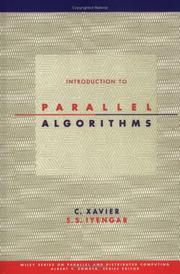## REVIEW - Introduction to Parallel AlgorithmsTitle:

Introduction to Parallel Algorithms

Author:

C. Xavier, S. S. Iyengar

ISBN:

Publisher:

John Wiley & Sons (1998)

Pages:

365pp

Reviewer:

Brian Bramer

Reviewed:

June 1999

Rating:

★★☆☆☆

Although sequential computer systems are becoming more powerful the problems faced in areas such as communications and multimedia systems are becoming more complex, e.g. real-time video compression/decompression, image manipulation, etc. Parallel systems are suited to such problems but the task of programming them in non-trivial. Many computing science courses now contain modules on parallel hardware and programming.

This book is in four parts. Part one lays the foundations starting with an overview of hardware such as pipelining, multiprocessing, etc., the classification of parallel systems (Flynn's, Erlangen, Duncan's, etc.) and models of parallel architectures (tree, hypercubes, network, etc.) then moving on the look at data structures (arrays, lists, graphs, etc.) and to consider suitable paradigms for parallel algorithms. Part two covers algorithms for graph models, part three array manipulation (searching and sorting) and part four parallel algorithms for numerical and computational methods (matrices, differentiation, integration and differential equations).

A text on a very specialised area of computing science. Worth considering as a set text for a module on parallel algorithms taken as part of a theoretical computing science course (a good mathematical foundation is assumed). Would enable workers starting a research programme in this area to gain a good foundation before moving on to a specialised topic. Note that the programming of algorithms is not covered.

Book cover image courtesy of Open Library.